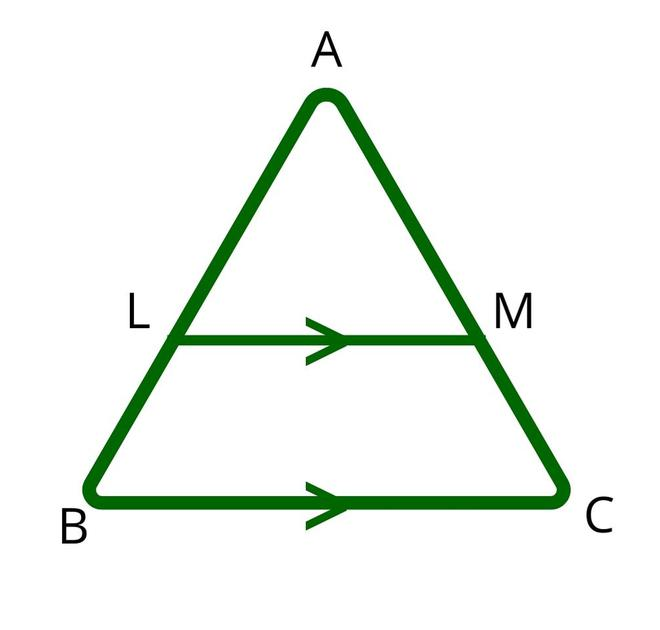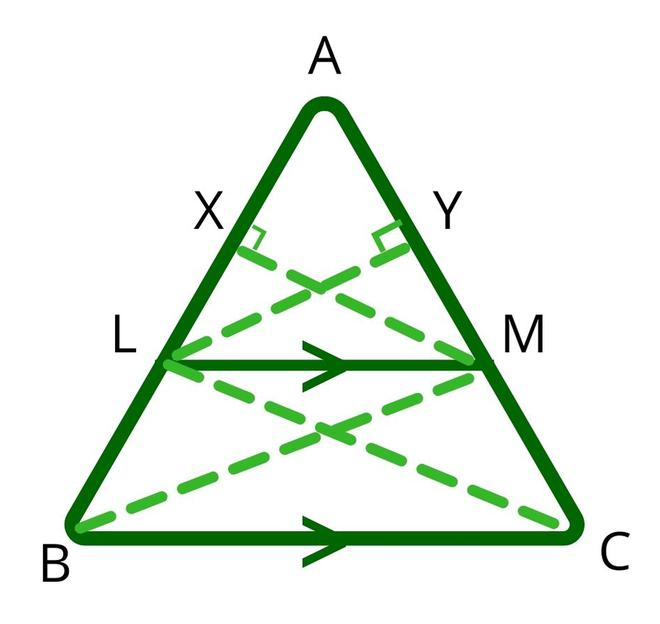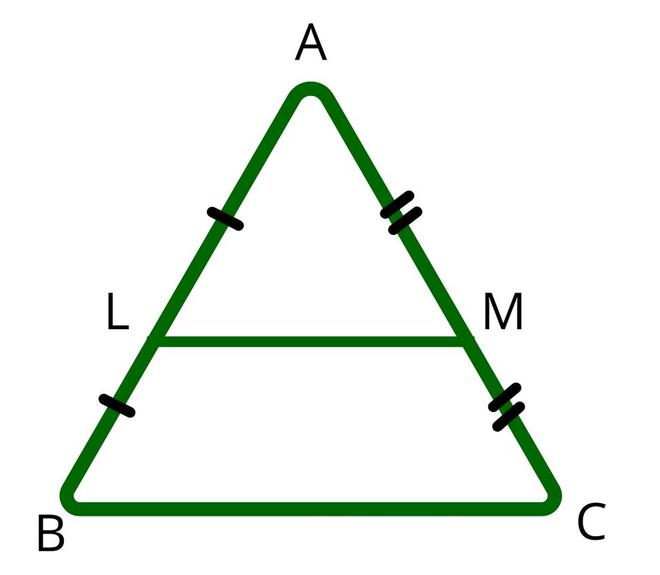Related Articles
Thales’s Theorem
• Last Updated : 19 Jan, 2021

### Proof of Basic Proportionality Theorem

Suppose we have a triangle ABC, if we draw a line LM parallel to side BC then the theorem states that,In triangle ABC, join the vertex B to M on line AC and join vertex C on line AB. Then, drop a perpendicular MX on line AB and perpendicular LY on AC. The below diagram shows the construction for the same.Since, area of triangle=Area ofALM=Area ofLBM=Area ofALM=Area ofLMC=Ratio of area ofALM andLBM:—-(1)

Ratio of area ofALM andLMC:—-(2)

According to the property of triangles, the triangles on the same base and between same parallel lines have equal areas.

Therefore,LBM andLMC have equal areas.—-(3)

From equations (1),(2), and (3) we can conclude:Hence, the basic proportionality theorem is proved.

### Solved Examples on Basic Proportionality Theorem

Example 1. In an ∆ABC, sides AB and AC  are intersected by a line at D and E respectively, which is parallel to side BC. Prove that AD/AB = AE/AC.

Solution:

Given: DE || BC. So, AD/DB = AE/EC

or By interchanging the ratios as => DB/AD = EC/AE

Now, add 1 on both sides=> (DB/AD)  + 1 = (EC/AE) + 1

If we interchange the ratios again, we get=> AD/AB = AE/AC

Hence, proved.

Example 2. In triangle ABC, where DE is a line drawn from the midpoint of AB and ends midpoint of AC at E. AD/DB = AE/EC and ∠ADE = ∠ACB. Then prove ABC is an isosceles triangle.

Solution: